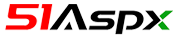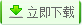| | 帮助?

[商业版 200RMB] WebForm,下载次数：1 次 | 关键字： PHP MYSQL OA 办公 网络办公## 源码目录树

;
```当前路径：Classes/PHPExcel/Worksheet/PageSetup.php
<?php
/**
* PHPExcel
*
* Copyright (c) 2006 - 2015 PHPExcel
*
* This library is free software; you can redistribute it and/or
* modify it under the terms of the GNU Lesser General Public
* version 2.1 of the License, or (at your option) any later version.
*
* This library is distributed in the hope that it will be useful,
* but WITHOUT ANY WARRANTY; without even the implied warranty of
* MERCHANTABILITY or FITNESS FOR A PARTICULAR PURPOSE.  See the GNU
* Lesser General Public License for more details.
*
* You should have received a copy of the GNU Lesser General Public
* License along with this library; if not, write to the Free Software
* Foundation, Inc., 51 Franklin Street, Fifth Floor, Boston, MA  02110-1301  USA
*
* @category   PHPExcel
* @package    PHPExcel_Worksheet
* @version    ##VERSION##, ##DATE##
*/

/**
* PHPExcel_Worksheet_PageSetup
*
* <code>
* Paper size taken from Office Open XML Part 4 - Markup Language Reference, page 1988:
*
* 1 = Letter paper (8.5 in. by 11 in.)
* 2 = Letter small paper (8.5 in. by 11 in.)
* 3 = Tabloid paper (11 in. by 17 in.)
* 4 = Ledger paper (17 in. by 11 in.)
* 5 = Legal paper (8.5 in. by 14 in.)
* 6 = Statement paper (5.5 in. by 8.5 in.)
* 7 = Executive paper (7.25 in. by 10.5 in.)
* 8 = A3 paper (297 mm by 420 mm)
* 9 = A4 paper (210 mm by 297 mm)
* 10 = A4 small paper (210 mm by 297 mm)
* 11 = A5 paper (148 mm by 210 mm)
* 12 = B4 paper (250 mm by 353 mm)
* 13 = B5 paper (176 mm by 250 mm)
* 14 = Folio paper (8.5 in. by 13 in.)
* 15 = Quarto paper (215 mm by 275 mm)
* 16 = Standard paper (10 in. by 14 in.)
* 17 = Standard paper (11 in. by 17 in.)
* 18 = Note paper (8.5 in. by 11 in.)
* 19 = #9 envelope (3.875 in. by 8.875 in.)
* 20 = #10 envelope (4.125 in. by 9.5 in.)
* 21 = #11 envelope (4.5 in. by 10.375 in.)
* 22 = #12 envelope (4.75 in. by 11 in.)
* 23 = #14 envelope (5 in. by 11.5 in.)
* 24 = C paper (17 in. by 22 in.)
* 25 = D paper (22 in. by 34 in.)
* 26 = E paper (34 in. by 44 in.)
* 27 = DL envelope (110 mm by 220 mm)
* 28 = C5 envelope (162 mm by 229 mm)
* 29 = C3 envelope (324 mm by 458 mm)
* 30 = C4 envelope (229 mm by 324 mm)
* 31 = C6 envelope (114 mm by 162 mm)
* 32 = C65 envelope (114 mm by 229 mm)
* 33 = B4 envelope (250 mm by 353 mm)
* 34 = B5 envelope (176 mm by 250 mm)
* 35 = B6 envelope (176 mm by 125 mm)
* 36 = Italy envelope (110 mm by 230 mm)
* 37 = Monarch envelope (3.875 in. by 7.5 in.).
* 38 = 6 3/4 envelope (3.625 in. by 6.5 in.)
* 39 = US standard fanfold (14.875 in. by 11 in.)
* 40 = German standard fanfold (8.5 in. by 12 in.)
* 41 = German legal fanfold (8.5 in. by 13 in.)
* 42 = ISO B4 (250 mm by 353 mm)
* 43 = Japanese double postcard (200 mm by 148 mm)
* 44 = Standard paper (9 in. by 11 in.)
* 45 = Standard paper (10 in. by 11 in.)
* 46 = Standard paper (15 in. by 11 in.)
* 47 = Invite envelope (220 mm by 220 mm)
* 50 = Letter extra paper (9.275 in. by 12 in.)
* 51 = Legal extra paper (9.275 in. by 15 in.)
* 52 = Tabloid extra paper (11.69 in. by 18 in.)
* 53 = A4 extra paper (236 mm by 322 mm)
* 54 = Letter transverse paper (8.275 in. by 11 in.)
* 55 = A4 transverse paper (210 mm by 297 mm)
* 56 = Letter extra transverse paper (9.275 in. by 12 in.)
* 57 = SuperA/SuperA/A4 paper (227 mm by 356 mm)
* 58 = SuperB/SuperB/A3 paper (305 mm by 487 mm)
* 59 = Letter plus paper (8.5 in. by 12.69 in.)
* 60 = A4 plus paper (210 mm by 330 mm)
* 61 = A5 transverse paper (148 mm by 210 mm)
* 62 = JIS B5 transverse paper (182 mm by 257 mm)
* 63 = A3 extra paper (322 mm by 445 mm)
* 64 = A5 extra paper (174 mm by 235 mm)
* 65 = ISO B5 extra paper (201 mm by 276 mm)
* 66 = A2 paper (420 mm by 594 mm)
* 67 = A3 transverse paper (297 mm by 420 mm)
* 68 = A3 extra transverse paper (322 mm by 445 mm)
* </code>
*
* @category   PHPExcel
* @package    PHPExcel_Worksheet
*/
class PHPExcel_Worksheet_PageSetup
{
/* Paper size */
const PAPERSIZE_LETTER                          = 1;
const PAPERSIZE_LETTER_SMALL                    = 2;
const PAPERSIZE_TABLOID                         = 3;
const PAPERSIZE_LEDGER                          = 4;
const PAPERSIZE_LEGAL                           = 5;
const PAPERSIZE_STATEMENT                       = 6;
const PAPERSIZE_EXECUTIVE                       = 7;
const PAPERSIZE_A3                              = 8;
const PAPERSIZE_A4                              = 9;
const PAPERSIZE_A4_SMALL                        = 10;
const PAPERSIZE_A5                              = 11;
const PAPERSIZE_B4                              = 12;
const PAPERSIZE_B5                              = 13;
const PAPERSIZE_FOLIO                           = 14;
const PAPERSIZE_QUARTO                          = 15;
const PAPERSIZE_STANDARD_1                      = 16;
const PAPERSIZE_STANDARD_2                      = 17;
const PAPERSIZE_NOTE                            = 18;
const PAPERSIZE_NO9_ENVELOPE                    = 19;
const PAPERSIZE_NO10_ENVELOPE                   = 20;
const PAPERSIZE_NO11_ENVELOPE                   = 21;
const PAPERSIZE_NO12_ENVELOPE                   = 22;
const PAPERSIZE_NO14_ENVELOPE                   = 23;
const PAPERSIZE_C                               = 24;
const PAPERSIZE_D                               = 25;
const PAPERSIZE_E                               = 26;
const PAPERSIZE_DL_ENVELOPE                    ...```

## 相关源码

Floor 5th,Daheng Building,Zhongguancun,Beijing,China,100080